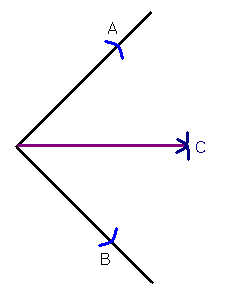## Ruler and Compass Constructions

You may need to know how to perform various constructions using a pair of compasses and an unmarked ruler (a ‘straight-edge’). When doing this sort of thing, you are not allowed to use any measuring equipment! You have to use the compasses to do the measuring...

You usually start by drawing a straight line with your ruler, or you might be given a straight line in an exam to start with.

Constructing an Equilateral Triangle

Suppose you have been given a line, AB, and you have to construct an equilateral triangle which has AB as one of it’s sides (an equilateral triangle is a triangle whose sides are all the same length).

Step 1: Put the compass point on A and open it so that the pencil touches B. So you have "measured" the length of AB with the compasses. Be careful not to open or close the compasses at all now!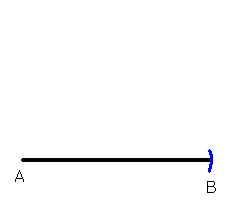Step 2: Leaving the compass point on A, draw an arc with the pencil roughly where you think the other vertex (corner) of the triangle is going to be. The distance from A to this arc is going to be the same as the length of AB. Therefore since we want to construct an equilateral triangle (with all sides the same length), our third vertex must be on the arc somewhere.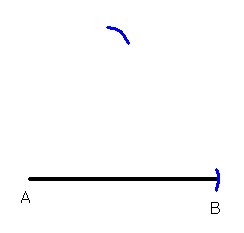Now move the compass point to B and draw another arc which crosses the first.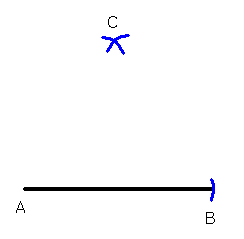Label where the two arcs cross C. Since the length of AC and BC are both equal to the length of AB, we have three points all the same distance from each other. If we join them up, we therefore have an equilateral triangle.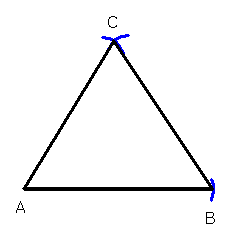The Midpoint of a Line Segment

Now suppose you are given a straight line and you have to find the midpoint.

Start in the same way as above, getting to the stage where you have found point C:Point C must be in the middle of A and B- it’s the same distance from A as it is from B, it’s just too high.

Construct another point D below the line AB, in exactly the same way as you constructed C.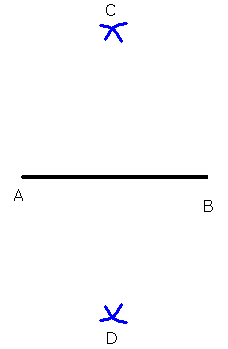Now we can join points C and D. The line CD is the perpendicular bisector of AB. This means it splits AB into two equal parts and also meets AB at right-angles. The midpoint of AB is where the two lines cross.

Bisecting an Angle

You may be given an angle and asked to bisect it (this means dividing it into two equal angles).

Start by opening your compasses to any length and drawing an arc on each line making up the angle. Label these points A and B.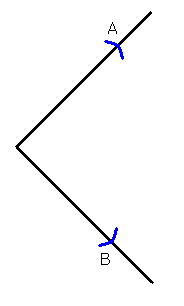Now, leaving the length of the compasses the same, put the compass point on A and draw an arc in the middle. Then put the compass point on B and draw an arc in the middle which crosses the first.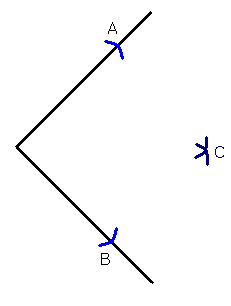This new point C will be in the middle of the angle. So we can draw a line from the angle to C and we will have split the angle in two.